• 多分类逻辑回归的基础概要和在Spark上的实现可参考： 多分类逻辑回归（Multinomial Logistic Regression） 多分类实现方式介绍和在Spark上实现多分类逻辑回归（Multinomial Logistic Regression） 本篇文章涉及到的...

个人网站：   文艺与Code | Thinkgamer的博客
CSDN博客：Thinkgamer技术专栏
知乎：          Thinkgamer
微博：          Thinkgamer的微博
GitHub：     Thinkgamer的GitHub
微信公众号：搜索与推荐Wiki

多分类逻辑回归基于逻辑回归（Logistic Regression，LR）和softMax实现，其在多分类分类任务中应用广泛，本篇文章基于tf实现多分类逻辑回归，使用的数据集为Mnist。

多分类逻辑回归的基础概要和在Spark上的实现可参考：

多分类逻辑回归（Multinomial Logistic Regression）
多分类实现方式介绍和在Spark上实现多分类逻辑回归（Multinomial Logistic Regression）

本篇文章涉及到的tf相关接口函数及释义如下：
tf.nn.softmax
Softmax 在机器学习和深度学习中有着非常广泛的应用。尤其在处理多分类（C > 2）问题，分类器最后的输出单元需要Softmax 函数进行数值处理。关于Softmax 函数的定义如下所示：
$S_i=\frac{e^{V_i}}{\sum_i^Ce^{V_i}}$
其中，Vi 是分类器前级输出单元的输出。i 表示类别索引，总的类别个数为 C。Si 表示的是当前元素的指数与所有元素指数和的比值。Softmax 将多分类的输出数值转化为相对概率，更容易理解和比较。我们来看下面这个例子。
一个多分类问题，C = 4。线性分类器模型最后输出层包含了四个输出值，分别是：
$V=\left[ \begin{matrix} -3 \\ 2 \\ -1 \\ 0 \end{matrix} \right]$
经过Softmax处理后，数值转化为相对概率：
$S=\left[ \begin{matrix} 0.0057 \\ 0.8390 \\ 0.0418 \\ 0.1135 \end{matrix} \right]$
很明显，Softmax 的输出表征了不同类别之间的相对概率。我们可以清晰地看出，S1 = 0.8390，对应的概率最大，则更清晰地可以判断预测为第1类的可能性更大。Softmax 将连续数值转化成相对概率，更有利于我们理解。
实际应用中，使用 Softmax 需要注意数值溢出的问题。因为有指数运算，如果 V 数值很大，经过指数运算后的数值往往可能有溢出的可能。所以，需要对 V 进行一些数值处理：即 V 中的每个元素减去 V 中的最大值。
$D=max(V)$$S_i=\frac{e^{V_i-D}}{\sum_i^Ce^{V_i-D}}$
tf中的softmax函数接口为：
tf.nn.softmax(
logits,
axis=None,
name=None,
dim=None
)


logits：非空张量
axis：将被执行的softmax纬度，默认为None
name：名称，默认为None
dim：axis的别名，默认为None

tf.log()
计算元素的自然对数
tf.reduce_sum()
求和
tf.reduce_mean()
求平均数
tf.argmax()
返回tensor 列表中最大值索引号，eg：

import tensorflow as tf
import numpy as np

A = [[1,3,4,5,6]]
B = [[1,3,4], [2,4,1]]

with tf.Session() as sess:
print(sess.run(tf.argmax(A, 1)))
print(sess.run(tf.argmax(B, 1)))

---------------------


[2 1]

tf.cast()
数据格式转换，eg：
a = tf.Variable([1,0,0,1,1])
b = tf.cast(a,dtype=tf.bool)
sess = tf.Session()
sess.run(tf.initialize_all_variables())
print(sess.run(b))

---------------------

[ True False False  True  True]

最终实现代码
# -*- coding: utf-8 -*-
"""
Author: Thinkgamer
Date: 2019-02-26
"""
import tensorflow as tf
from tensorflow.examples.tutorials.mnist import input_data

# 加载mnist数据集
train_img = mnist.train.images
train_label = mnist.train.labels
test_img = mnist.test.images
test_label = mnist.test.labels

print("Mnist数据集加载成功！")
print(train_img.shape)
print(train_label.shape)
print(test_img.shape)
print(test_label.shape)
print(train_label)

# 构建模型计算图
x = tf.placeholder(dtype=float, shape=[None, 784])
y = tf.placeholder(dtype=float, shape=[None, 10])
w = tf.Variable(tf.zeros([784,10]))
b = tf.Variable(tf.zeros())

actv = tf.nn.softmax(tf.matmul(x,w) + b)
cost = tf.reduce_mean(-tf.reduce_sum(y * tf.log(actv),reduction_indices=1))
learning_ratio = 0.01

# 预测值
pred = tf.equal(tf.argmax(actv, 1), tf.argmax(y, 1))
# 精确值
accr = tf.reduce_mean(tf.cast(pred, "float"))
# 初始化
init = tf.global_variables_initializer()

# 开始训练模型
training_epochs = 50
batch_size      = 100
display_step    = 5

with tf.Session() as sess:
sess.run(init)
# 小批量梯度下降算法优化
for epoch in range(training_epochs):
avg_cost = 0.
num_batch = int(mnist.train.num_examples/batch_size)
for i in range(num_batch):
batch_xs, batch_ys = mnist.train.next_batch(batch_size)
sess.run(optm, feed_dict={x: batch_xs, y: batch_ys})
feeds = {x: batch_xs, y: batch_ys}
avg_cost += sess.run(cost, feed_dict=feeds)/num_batch

# DISPLAY
if epoch % display_step == 0:
feeds_train = {x: batch_xs, y: batch_ys}
feeds_test = {x: mnist.test.images, y: mnist.test.labels}
train_acc = sess.run(accr, feed_dict=feeds_train)
test_acc = sess.run(accr, feed_dict=feeds_test)
print ("Epoch: %03d/%03d cost: %.9f train_acc: %.3f test_acc: %.3f"% (epoch, training_epochs, avg_cost, train_acc, test_acc))
print ("DONE")

运行代码输出为：
Mnist数据集加载成功！
(55000, 784)
(55000, 10)
(10000, 784)
(10000, 10)
[0. 0. 0. 0. 0. 0. 0. 1. 0. 0.]

Epoch: 000/050 cost: 1.176693809 train_acc: 0.890 test_acc: 0.856
Epoch: 005/050 cost: 0.440978021 train_acc: 0.870 test_acc: 0.895
Epoch: 010/050 cost: 0.383333536 train_acc: 0.900 test_acc: 0.904
Epoch: 015/050 cost: 0.357253272 train_acc: 0.970 test_acc: 0.909
Epoch: 020/050 cost: 0.341492714 train_acc: 0.910 test_acc: 0.913
Epoch: 025/050 cost: 0.330541673 train_acc: 0.920 test_acc: 0.915
Epoch: 030/050 cost: 0.322343116 train_acc: 0.910 test_acc: 0.915
Epoch: 035/050 cost: 0.315980227 train_acc: 0.900 test_acc: 0.917
Epoch: 040/050 cost: 0.310762640 train_acc: 0.910 test_acc: 0.918
Epoch: 045/050 cost: 0.306386642 train_acc: 0.900 test_acc: 0.919
DONE

Process finished with exit code 0


从最终的结果可以看出：

loss一直在下降，说明模型是拟合的
训练集和测试机的精确率都很高，模型效果表现不错
当然也可以通过调节batch_size，epochs等参数进行效果调优

【技术服务】，详情点击查看：
https://mp.weixin.qq.com/s/PtX9ukKRBmazAWARprGIAg扫一扫 关注微信公众号！号主 专注于搜索和推荐系统，尝试使用算法去更好的服务于用户，包括但不局限于机器学习，深度学习，强化学习，自然语言理解，知识图谱，还不定时分享技术，资料，思考等文章！


展开全文Tensorflow Mnist
• 13. 使用逻辑回归进行多分类(with codes) 逻辑回归也可以进行多分类任务。 from sklearn.datasets import make_classification from sklearn.linear_model import LogisticRegression from sklearn.model_...
13. 使用逻辑回归进行多分类(with codes)
逻辑回归也可以进行多分类任务。
from sklearn.datasets import make_classification
from sklearn.linear_model import LogisticRegression
from sklearn.model_selection import train_test_split

# n_clusters_per_class 每个类别生成的簇的数量。
X, y = make_classification(n_samples=200, n_features=2, n_informative=2, n_redundant=0,
n_classes=3, random_state=0, n_clusters_per_class=1)
X_train, X_test, y_train, y_test = train_test_split(X, y, test_size=0.25, random_state=0)
# lr = LogisticRegression()
# lr = LogisticRegression(multi_class="ovr")
# 多分类任务可以使用"ovr"（一对其他）与multinomial（多项式）来进行处理。
# 如果使用多项式进行处理，则solver（解决方案）不能使用默认的liblinear。
lr = LogisticRegression(multi_class="multinomial", solver="lbfgs")
lr.fit(X_train, y_train)
# 在多分类时，默认使用的方式为ovr，通过多个二分类的组合，实现多分类的任务。
# 在多分类情况下，模型的权重与偏置分为n组（内部为维护n个二分类的模型），其中n为分类的数量。
print(f"模型权重：{lr.coef_}")
print(f"模型偏置：{lr.intercept_}")
y_hat = lr.predict(X_test)
print(f"分类正确率：{lr.score(X_test, y_test):.3f}")
print(lr.predict_proba(X_test[:5]))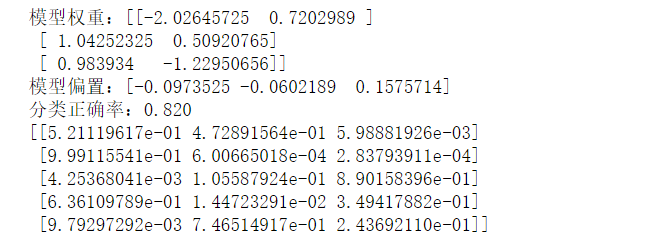import numpy as np
import matplotlib as mpl
import matplotlib.pyplot as plt
from matplotlib.colors import ListedColormap

mpl.rcParams["font.family"] = "SimHei"
mpl.rcParams["axes.unicode_minus"] = False

cmap = ListedColormap(["r", "g", "b"])
marker = ["o", "v", "x"]

x1_min, x2_min = np.min(X_test, axis=0)
x1_max, x2_max = np.max(X_test, axis=0)
x1 = np.linspace(x1_min - 1, x1_max + 1, 100)
x2 = np.linspace(x2_min - 1, x2_max + 1, 100)
X1, X2 = np.meshgrid(x1, x2)
Z = lr.predict(np.array([X1.ravel(), X2.ravel()]).T).reshape(X1.shape)
plt.contourf(X1, X2, Z, cmap=cmap, alpha=0.5)
for i, class_ in enumerate(np.unique(y_test)):
plt.scatter(x=X_test[y_test == class_, 0], y=X_test[y_test == class_, 1],
c=cmap(i), label=class_, marker=marker[i])
plt.legend()
plt.show()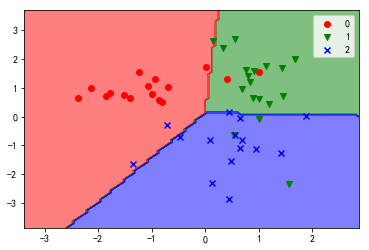展开全文• 1. 为什么不选用线性回归 线性回归并不适用于定性预测...2.逻辑回归逻辑回归对目标值属于某个类别建模 P(Y=C|X1,X2...Xn)P(Y=C|X_1,X_2...X_n) 如何对p(X)=Pr(Y=c|X)p(X) = Pr(Y = c|X)建模？ 在线性回归的基础上引
1. 为什么不选用线性回归

线性回归并不适用于定性预测值的预测
即使在二元分类中利用哑变量对类别编码，其预测的值也会不落在[0,1]，这样就很难利用概率的方式来解释

2.逻辑回归

逻辑回归对目标值属于某个类别建模 P(Y=C|X1,X2...Xn)<!--//--><![CDATA[//><!--
P(Y=C|X_1,X_2...X_n)
//--><!]]>
如何对p(X)=Pr(Y=c|X)<!--//--><![CDATA[//><!--
p(X) = Pr(Y = c|X)
//--><!]]>建模？
在线性回归的基础上引入逻辑函数，将预测值限制在（0,1）之间 p(X)=eβ0+β1X1+eβ0+β1X<!--//--><![CDATA[//><!--
p(X) = \frac {e^{\beta_0 + \beta_1 X}} {1 + e^{\beta_0 + \beta_1 X}}
//--><!]]>
利用最大似然方法拟合该模型
odds：p(x)1−p(x)=eβ0+β1X<!--//--><![CDATA[//><!--
\frac {p(x)} {1-p(x)} = e^{\beta_0 + \beta_1 X}
//--><!]]>
log-odds：log(p(x)1−p(x))=β0+β1X<!--//--><![CDATA[//><!--
log(\frac {p(x)} {1-p(x)})=\beta_0 + \beta_1 X
//--><!]]>
经过简单的数学变化，可以获得odds和log-odds，odd反应了属于c类的可能性，log-odds反应了逻辑回归模型和线性回归模型的关系，虽然log-odds和X不是线性增长的关系，但X增加和log-odds也呈现了正相关。

3.参数估计

同线性回归模型一样，逻辑回归模型中β0<!--//--><![CDATA[//><!--
\beta_0
//--><!]]>和β1<!--//--><![CDATA[//><!--
\beta_1
//--><!]]>并不可知，需要通过训练集来模拟，在线性回归中采用了最小二乘法来拟合数据，但逻辑回归通常采用最大似然估计

4.多元逻辑回归

log(p(x)1−p(x))=β0+β1X1+...+βnXp<!--//--><![CDATA[//><!--
log(\frac {p(x)} {1-p(x)})=\beta_0 + \beta_1 X_1+...+\beta_n X_p
//--><!]]>
注意分析特征之间的相关性

5.多类别逻辑回归

例如当数据可以划分为c1;c2;c3;<!--//--><![CDATA[//><!--
c_1;c_2;c_3;
//--><!]]>。我们希望对Pr(Y=c1|X);Pr(Y=c2|X)<!--//--><![CDATA[//><!--
Pr(Y = c_1|X);Pr(Y=c_2|X)
//--><!]]>同时保证Pr(Y=c3|X)=1−Pr(Y=c1|X)−Pr(Y=c2|X)<!--//--><![CDATA[//><!--
Pr(Y=c_3|X)=1-Pr(Y = c_1|X)-Pr(Y=c_2|X)
//--><!]]>多类别的逻辑回归效果并不好，线性判别分析是更为流行的多类别分类问题

逻辑回归方法是线性回归模型的拓展，参数式的学习方法更容易理解和解释，逻辑回归适用于二类分类，容易实现和解释。

逻辑回归实例
展开全文• 其中二分类是最常见且使用最多的分类场景，解决二分类的算法有很，比如： 基本的KNN、贝叶斯、SVM Online Ranking中用来做二分类的包括FM、FFM、GBDT、LR、XGBoost等 多分类中比如： 改进版...
 前言

分类从结果的数量上可以简单的划分为：

二分类（Binary Classification）
多分类（Multinomial  Classification）。
其中二分类是最常见且使用最多的分类场景，解决二分类的算法有很多，比如：

基本的KNN、贝叶斯、SVM
Online Ranking中用来做二分类的包括FM、FFM、GBDT、LR、XGBoost等
多分类中比如：

改进版的KNN、改进版的贝叶斯、改进版的SVM等
多类别的逻辑回归
啰嗦了这么多，其实就是为了说这个多分类的逻辑回归。

简介

在统计学里，多类别逻辑回归是一个将逻辑回归一般化成多类别问题得到的分类方法。用更加专业的话来说，它就是一个用来预测一个具有类别分布的因变量不同可能结果的概率的模型。

另外，多类别逻辑回归也有很多其它的名字，包括polytomous LR，multiclass LR，softmax regression，multinomial logit，maximum entropy classifier，conditional maximum entropy model。

在多类别逻辑回归中，因变量是根据一系列自变量（就是我们所说的特征、观测变量）来预测得到的。具体来说，就是通过将自变量和相应参数进行线性组合之后，使用某种概率模型来计算预测因变量中得到某个结果的概率，而自变量对应的参数是通过训练数据计算得到的，有时我们将这些参数成为回归系数。

模型分析

1、线性分类器

多分类逻辑回归使用的是跟线性回归一致的线性预测函数，其基本表达式如下：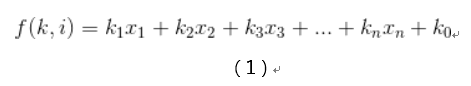其中i=[1,n]这里的k是一个回归系数，它表示的是第n个观测变量/特征对地n个结果的影响有多大。这里将1看做x0，我们可以得到上述公式的向量化形式：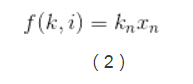这里kn是一个回归系数向量，表示的是观测向量xi表示的观测数据对结果k的影响度，或者叫重要性。

2、逻辑回归

多分类逻辑回归是基于逻辑回归（Logistic Regression）来做的，逻辑回归的基本表示如下：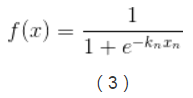其中y=1时，f（x）的表达式为：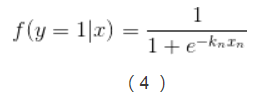则y=0时，f（x）的表达式为：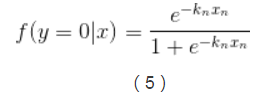3、k-1个独立二元逻辑回归到多分类逻辑回归的扩展

实现多类别逻辑回归模型最简单的方法是，对于所有K个可能的分类结果，我们运行K−1个独立二元逻辑回归模型，在运行过程中把其中一个类别看成是主类别，然后将其它K−1个类别和我们所选择的主类别分别进行回归。通过这样的方式，如果选择结果K作为主类别的话，我们可以得到以下公式。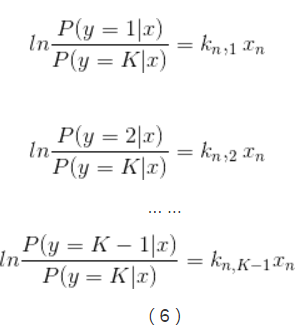其推导过程如下：

这里有个假设的前提：y=K-1和y=K的概率和为1，即：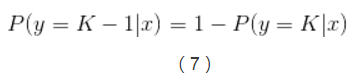将逻辑回归的表达式带入可得：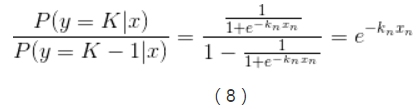公式两边同时求ln，可得：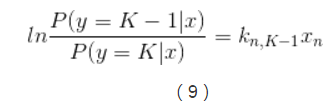在公式（6）中已经引入了所有可能的回归系数集合，对公式（6）两边进行指数化处理，能够得到以下公式：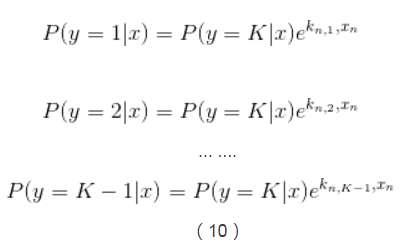因为所有概率的和为1，所以可以得到：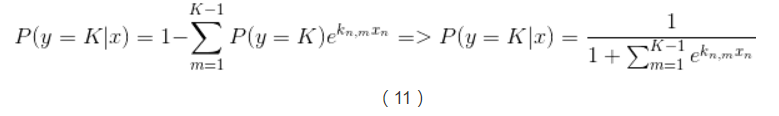这样我们就能计算出所有给定未预测样本情况下某个结果的概率，如下：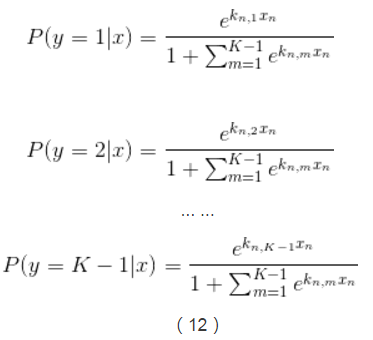回归参数的估计

上面篇幅所涉及到的每一个权重向量kn中的未知系数我们可以通过最大后验估计（MAP）来计算，同时也可以使用其它方法来计算，例如一些基于梯度的算法。

二元逻辑回归对数模型到多分类逻辑回归的扩展

在上文中提到了由K-1个独立二元回归到多分类逻辑回归的扩展，这里介绍另外一种多分类逻辑回归的扩展——使用线性预测器和额外的归一化因子（一个配分函数的对数形式）来对某个结果的概率的对数进行建模。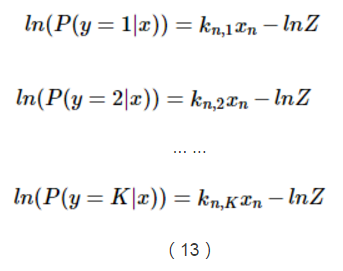这里用一个额外项-ln(Z)来确保所有概率能够形成一个概率分布，从而使得这些概率的和等于1。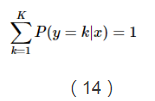然后将等式两边的进行指数化，我们可以得到以下公式：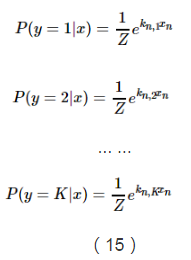由于上面说到，所有概率之和等于1，因此我们可以得到Z的推导公式：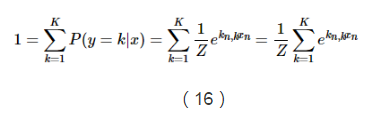通过上边的公式进行计算，可得：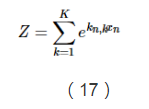综合以上的公式，我们最后可以得到每一个结果对应的概率公式：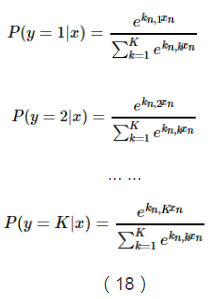仔细观察的话，我们可以发现，所有的概率都具有以下形式：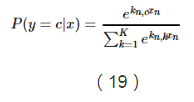我们可以把具有以下形式的函数成为softMax函数：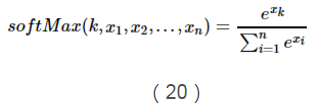这个函数能够将x1,...,xn之间的差别放大，当存在一个xk比所有值中的最大值要小很多的话，那么它对应的softMax函数值就会区域0。相反，当xk是最大值的时候，除非第二大的值跟它很接近，否则的话softMax会趋于1。所以softmax函数可以构造出一个像是平滑函数一样的加权平均函数。

所以，我们可以把上面的概率公式写成如下softMax函数的形式：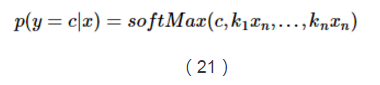至此，多分类的逻辑回归形式以及介绍完了，后续会进行最大似然函数的学习，敬请期待！扫一扫 关注微信公众号！号主 专注于搜索和推荐系统，尝试使用算法去更好的服务于用户，包括但不局限于机器学习，深度学习，强化学习，自然语言理解，知识图谱，还不定时分享技术，资料，思考等文章！

【技术服务】，详情点击查看：https://mp.weixin.qq.com/s/PtX9ukKRBmazAWARprGIAg


展开全文• 目录二分类逻辑回归数据准备模型构建模型检验多分类逻辑回归分类逻辑回归 首先，我先展示下我逻辑回归的总体代码，如果有基础的同志需要的话，可以直接修改数据和参数拿去用呀： library(lattice) library(ggplot...r语言
• 本文介绍回归模型的原理知识，包括线性回归、多项式回归和逻辑回归，并详细介绍Python Sklearn机器学习库的LinearRegression和LogisticRegression算法及回归分析实例。进入基础文章，希望对您有所帮助。机器学习
• http://blog.csdn.net/programmer_wei/article/details/52072939Logistic Regression（逻辑回归）是机器学习中一个非常非常常见的模型，在实际生产环境中也常常被使用，是一种经典的分类模型（不是回归模型）。...
• 【阿里天池云-龙珠计划】python机器学习小记 <task01： 基于sklearn的Logistic Regression分类实现>...逻辑回归（Logistic regression，简称LR）是一个分类模型，并且广泛应用于各个领域之中。 其最为突出的两点python 算法 机器学习
• 逻辑回归主要用来解决二分类问题，但是在工作学习中，我们肯定会碰到多分类的问题，下面就简单介绍下用逻辑回归解决多分类问题的方法。 one-VS-rest 从上面的名字就可以看出来，这个做法是通过对一个n分类问题训练n...
• 逻辑回归(Logistic Regression, LR)是传统机器学习中的一种分类模型，由于算法的简单和高效，在实际中应用非常广泛。它的起源非常复杂，可以看参考引用1。具体应用实践可以看这里。 问题背景 对于二元分类问题，...机器学习 LR
• 对于逻辑回归模型的相关知识点的总结与整理 简单描述一下逻辑回归的思想，逻辑回归是什么？ 逻辑回归虽然叫回归，但是其实是一种分类机器学习算法，原理是将数据拟合到一个预测logistic函数中，预测logistic函数...机器学习
• 逻辑回归知识要点一、逻辑回归模型模型简介：算法的分类思想算法模型sigmoid函数函数原型sigmoid函数图像参数求解二、逻辑回归实现二分类模型训练与预测结果可视化计算概率值绘制决策边界三、逻辑回归实现多分类建模...python 机器学习
• 逻辑回归模型分析步骤展示 参数 --- data:DataFrame,建模数据 """ data = transLabel(data) visualData(data) analyseData(data) #将数据分为训练集和测试集 trainSet,testSet = train_test_split(data,...Python 数据科学
• 主要介绍了二元分类及多元分类中的逻辑回归模型的假设函数、代价函数、优化算法及其他的一些细节问题。机器学习基础
• ## 逻辑回归模型

千次阅读 2018-04-08 13:39:02
本文作为美团机器学习InAction系列中的一篇，主要关注逻辑回归算法的数学模型和参数求解方法，最后也会简单讨论下逻辑回归和贝叶斯分类的关系，以及在多分类问题上的推广。逻辑回归 问题 实际工作中，我们可能会...
• 在一元线性回归模型一文中提到过很机器学习模型都是在线性回归模型的基础上创建的，本篇文章就简单的描述一下如何将线性回归应用到分类问题中——即逻辑回归逻辑回归的基本概念 线性回归针对的是连续型的数据...机器学习
• 通常，我在回归类中一直说的一句话是“请，看看您的数据”。在上一篇文章中，我们一直像大多数计量经济学家一样玩：我们没有查看数据。实际上，如果我们查看单个损失的分布，那么在数据集中，我们会看到以下内容： ...R语言
• 数据集Car（1728个观测值，6个自变量，因变量Car有unacc\acc\good\very good.../*逻辑回归数据集Car（1728个观测值，每个含6个属性，目标变量Car(unacc\acc\good\very good)）*/ /*导入数据集australian到逻辑库work中
• 之前对这个没有了解过，所以接下来需要花点时间学习这个模块了，今天主要是简单地基于官方给出来实例来实践一下逻辑回归分类模型，pyspark提供的逻辑回归分类模型主要包括：二项逻辑回归逻辑回归，各自有...
• ## 解析逻辑回归模型

千次阅读 2018-06-18 11:33:24
在模型层面上，逻辑回归模型是被用来解决分类问题的。由于分类是一个非线性问题，所以建模的主要难点是如何将非线性问题转化为线性问题。主要从两方面入手： - 从分解问题的角度入手：通过引入隐含变量（这里举一...ROC曲线
• 对于多分类模型，我们将简单介绍Spss中的多分类线性判别分析多分类逻辑回归的操作步骤 利用spass软件对数据进行预处理：生成虚拟变量 1.生成虚拟变量 2.删除多余虚拟变量 3.修改虚拟变量的名称 二、逻辑回归的...
• 逻辑回归模型逻辑回归属于机器学习中的较为经典的模型，在很金融专业的实证部分都会用到逻辑回归。接下来分成以下几个部分对逻辑回归模型进行介绍。1. 模型简介逻辑回归中的“逻辑”一次，并不是突出这个算法的...
• 构建一个逻辑回归分类器来进行图像二分类 逻辑回归原理介绍 逻辑回归主要用来处理二分类问题，目的是预测当前被观察的对象属于哪个组。它会给你提供一个离散的二进制(0或者1)输出结果。逻辑回归的实现过程主要...图像识别
• 分类选择模型 当被解释变量Y为 取有限个可能值的分类变量时，需要建立分类选择模型。分类选择模型大约有十几个左右，例如： 线性概率模型 ... 逻辑回归模型 条件逻辑回归模型 名...logistic ROC lift曲线 KS曲线
• 利用Scikit-Learn对数据进行逻辑回归分析 ...表现在随机逻辑回归模型上（书上程序中使用的） 对训练数据进行次采样拟合回归模型，即在不同的数据子集和特征子集上运行特征算法，不断重复，最终选择得分高的重要...
• 目录 逻辑回归-分类算法 1 概述 2sigmoid函数 3 逻辑回归公式 4 逻辑回归的损失函数 5 均方误差与对数自然损失对比 6sklearn逻辑回归API 7 案例 8 总结 逻辑回归-分类算法 1...损失函数 机器学习
• 下面介绍下利用sklearn做逻辑回归模型 做模型一般分为:提取数据---->了解数据(所谓的探索性数据)---->数据预处理(包括但不限于填充缺失值，特征提取，转换哑变量)---->选择模型---->验证模型---->...机器学习 人工智能
• 一、逻辑斯蒂分布/回归模型 模型描述的是一种什么样的事件或现象： 设X是连续随机变量，X服从逻辑斯蒂回归分布是指X具有下列分布函数和密度函数： 附上逻辑斯蒂分布的密度函数与分布函数，如下： 物理...
• 所以逻辑回归只能解决二分类问题，那么逻辑回归该如何解决多分类问题呢？ ** OVR OVR（One Vs Rest） OVR的思想 : 还是讲类别分为两类: 一类是A类，另一类就是其他所有类（把其他所有类当成一类来看），如图: 上图...机器学习
• 逻辑回归模型是一个非常非常非常常用的模型，在介绍LR之前，先介绍下广义线性模型1. 广义线性模型机器学习中常用于分类的“广义线性模型”，即对y进行变换使其为输入的线性叠加，注意g必须是单调可微的2. 逻辑回归...LR...